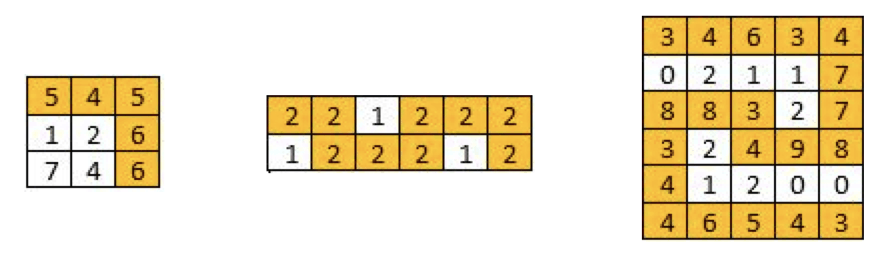# 1102. Path With Maximum Minimum Value

Reference: LeetCode
Difficulty: Medium

## Problem

Given a matrix of integers A with R rows and C columns, find the maximum score of a path starting at [0, 0] and ending at [R-1, C-1].

The score of a path is the minimum value in that path. For example, the value of the path 8 → 4 → 5 → 9 is 4.

A path moves some number of times from one visited cell to any neighbouring unvisited cell in one of the 4 cardinal directions (north, east, west, south).

Note:

• 1 <= R, C <= 100
• 0 <= A[i][j] <= 10^9

Example:## Analysis

### Backtracking

Here is the code of backtracking Time Limit Exceeded.

Time: $O(4^{RC})$
Space: $O(RC)$

### Priority Queue

Use a max heap. Consider the following example.

Notice that in the above example it stops expanding at 5 -> 4 since there is a better way to go.

Note:

• Learn how to write the comparator.
• Learn how to manage four-direction traversal.

Time: $O(RC \log{(RC)})$
Space: $O(RC)$

## Two-Direction Version

Given a matrix with r rows and c columns, find the maximum score of a path starting at [0, 0] and ending at [r - 1, c - 1]. The score of a path is the minimum value in that path. For example, the score of the path 8 → 4 → 5 → 9 is 4.

Don’t include the first or final entry. You can only move either down or right at any point in time.

Playground: https://leetcode.com/playground/sbkFjCbE

### DP

Define: dp[i][j] is the max score from  to [i][j]
Recurrence: dp[i][j] = max( min(dp[i-1][j], grid[i][j]), min(dp[i][j-1]), grid[i][j] )

#### DP (2D)

Time: $O(rc)$
Space: $O(rc)$

#### DP (1D)

Time: $O(rc)$
Space: $O(r)$ or $O(c)$

CommentJunhao Wang
a software engineering cat